Need Help?

Subscribe to Probability

###### \${selected_topic_name}
• Notes
• Comments & Questions

The length of an injection-molded plastic case that
holds magnetic tape is normally distributed with a length of
90.2 millimeters and a standard deviation of 0.1 millimeter.

(a) What is the probability that a part is longer than $90.3 \mathrm{mil}-$
limeters or shorter than 89.7 millimeters?
(b) What should the process mean be set at to obtain the great-
est number of parts between 89.7 and 90.3 millimeters?
(c) If parts that are not between 89.7 and 90.3 millimeters are
scrapped, what is the yield for the process mean that you
selected in part (b)?

$\mu=90.2 \mathrm{mm}$

$\sigma=0.1$

(a) $P(x>90.3)+P(x<89.7)$

$Z=\frac{x-\mu}{\sigma}$

$P\left(Z>\frac{90.3-90.2}{0.1}\right)+P\left(Z<\frac{89.7-90.2}{0.1}\right)$

$=P(Z>1)+P(Z<-5)$

$=1-P(Z<1)+P(Z<-5)$

$\Rightarrow\left(\phi{1}\right), \phi(-5)$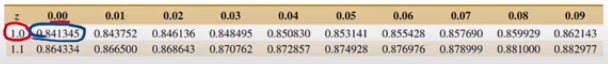$=1.841345+0=0.15866$

(b) $\mu=\frac{90.3-89.7}{2}=90$

(c) $\mu=90$

$P(89.7<x<90.3)\mathrm{m}$

$=P\left(\frac{89.7-90}{0.1}<Z<\frac{90.3-90}{0.1}\right)$

$=P(-3<Z<3) \rightarrow \phi(-3), \phi(3)$

$=P\left(Z<3\right)-P(Z<-3)=\phi(3)-\phi(-3)$

$=0.998650-0.001350=0.9973=99.73 \%$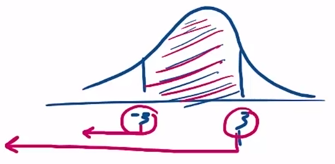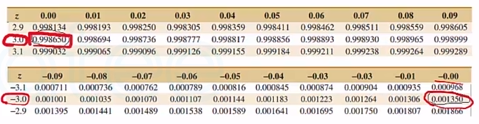The diameter of the dot produced by a printer is normally distributed with
a mean diameter of 0.002 inch and a standard deviation of 0.0004 inch.
(a) What is the probability that the diameter of a dot exceeds
0.0026 inch?

(b) What is the probability that a diameter is between 0.0014
and 0.0026 inch?
(c) What standard deviation of diameters is needed so that the
probability that the diameter of a dot does not exceed 0.0025 inch is 0.9049 ?

$\mu=0.002 \mathrm{inch}$
$\sigma=0.0004 \mathrm{inch}$

(a) $P(x>0.0026)$

$=P\left(Z>\left(\frac{0.0026-0.002}{0.0004}\right)\right)$

$=P(Z>1.5)$

$=1-P(Z<1.5)$

$=1-\phi(1.5)=1-0.933193=0.06681$

(b) $P(0.0014<x<0.0026)$

$P\left(\frac{0.0014-0.002}{0.0004}<Z<\frac{0.0026-0.002}{0.0004}\right)$

$P(-1.5<Z<1.5)$

$=P(Z<1.5)-P(Z<-1.5)=\phi(1.5)-\phi(-1.5)$

$=0.933193-0.066807=0.86639$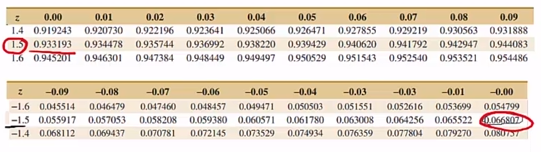(c) $\sigma ? !$

If $P(x<0.0025)=0.9049$

$P\left(Z<\frac{0.0025-0.002}{\sigma}\right)=0.9049$

$Z=1.31$

$=\frac{0.0025-0.002}{\sigma}=0.00038$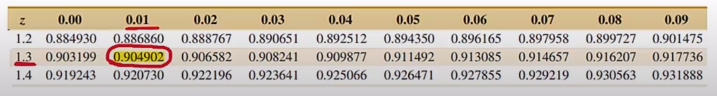No comments yet